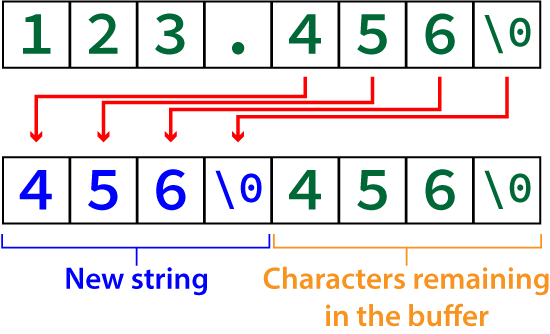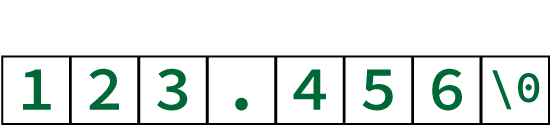# From Decimal Value to a String

The challenge for this month’s Exercise is to split a decimal value into its integer and fractional portions. But what if you need the fractional portion as a string?

It’s uncomplicated to use math to cleave the integer and decimal portion of a value: Changing the data type of float 123.456 into an int creates 123. The rest of the operation is just math.

Splitting the value as a string involves converting the real number into a string, then hacking off the integer portion or any text to the left of — and including — the decimal. So the string:

`"123.456"`

Becomes:

`"456"`

The function that does the heavy lifting here is snprintf(). This cousin to printf() sends its output to a buffer instead of standard output. The n in the function name indicates the buffer size, which makes this cousin better than the sprintf() function it replaces. The only caveat is that the function is non-standard, so it’s unavailable in some compilers. (Read more about snprintf() here.)

To place the decimal value `a` into char `buffer` with length `size`, the following statement is used:

`snprintf(buffer,size,"%f",a);`

Once the float value is converted into a string, a loop processes the characters, plucking out only those that follow the decimal. Two buffers are used for this process, as illustrated in Figure 1.Figure 1. Copying the fractional portion into a new buffer.

Another approach, one that my memory-stingy programmer upbringing encourages me to try, is to copy the data within the same buffer. After all, the string’s decimal portion is unnecessary, and the `%f` conversion character is configured by default to prefix `0.` to any fractional value. This approach is illustrated in Figure 2.Figure 2. Copying the fractional portion within the same buffer.

The following code includes the decimal() function, which returns a string representing the decimal portion of an input value. It uses the technique illustrated in Figure 2 to process the string.

### 2022_05_14-Lesson.c

```#include <stdio.h>

char *decimal(float a)
{
const int size=32;
static char buffer[size];        /* more than enough room */
int offset,base;

snprintf(buffer,size,"%f",a);
offset = base = 0;
/* first, locate the decimal */
while( buffer[offset]!='.' )
{
offset++;
}
/* copy the characters to the front */
offset++;        /* skip over the decimal */
while( buffer[offset] )
{
buffer[base] = buffer[offset];
offset++;
base++;
}
buffer[base] = '\0';    /* cap the string */

return(buffer);
}

int main()
{
float a;

printf("Enter a decimal value: ");
scanf("%f",&a);

printf("Decimal portion is: %s\n",decimal(a));

return(0);
}```

Variable `buffer` is declared as static at Line 6 to ensure that its contents aren’t discarded when the function returns. The snprintf() function at Line 9 converts float value `a` into a string in `buffer`.

The while loop at Line 12 locates the decimal within the string, the dividing character between the value’s integer and fractional part.

A second while loop at Line 18 moves characters from the fractional part forward.

Line 24 caps the new string, truncating the old string, as illustrated in Figure 2.

Here’s a sample run:

```Enter a decimal value: 123.456 Decimal portion is: 456001```

A problem occurs due to precision, as the decimal portion .456 appears as 0.456001. I suppose you could deal with the digital detritus, though doing so it’s part of this code. Effectively, the decimal() function returns the fractional part of a real number as a string.

## 3 thoughts on “From Decimal Value to a String”

1.ivanko13 on said:

Trailing 001 can be omited with conversion specificator %g. The GNU C Library Reference Manual states that for handeling floating-point numbers conversion specificator %g, %G uses either %e or %f format , depending on what is more appropriet for the magnitude of the particular number. I can’t explain why the number is printed without trailing 001.

2.ivanko13 on said:

It seems to round to three decimal places. Not helpful in your example.

3.dgookin on said:

That’s a nifty trick. Thanks!